# Final Lab Report[TYPE TITLE HERE IN CAPS INCLUDING MODULE NUMBER]
[Type Name Here]
EE1305/EE1105 - Intro to EE
The University of Texas at El Paso
[Type Due Date Here]
Lab Objectives
1. Explaining the concept of operation of op-amp
2. Determine the operation of op-amp
3. Using the oxygen sensor in an experiment
4. Plotting filter signals of an op-amp
5. Analyzing the filter signals
6. Showing the objective use of the op-amp
I certify that I am the creator of all the written documentation submitted as part of this
report, that all data and illustrations are original and created by me, and that I have not
shared any part of this report with any of my fellow engineering students. REPORTS
NOT SIGNED WILL NOT BE GRADED.
Electronic Signature: _______________________________________
LAB: ____
TEAM: ____
I. Introduction
In the set up done in the lab, an oxygen sensor was used to measure the pulse of a human body in
the lab. The oxygen sensor is usually an electronic device that can be used to measure the
amount of oxygen gas being analyzed. It was developed by a scientist known as Robert Bosch in
the period of late 1960s who was supervised by his supervisor in the lab called Dr. Gunter
Bauman. The original sensing organ used by this scientist was made up from a thimble ceramic
coated up on both sides using a thin layer of platinum and was in both heated and non-heated
form. The currently used sensor made of planar-style was brought up in the 1990s and has a
reduced ceramic and responds faster. In this lab, it was used to measure the proportion of oxygen
amount in the body of a human being. Scientist uses oxygen sensors in a different approach like
as the oxygen analyzers which is most applicable in medicine especially in anesthesia monitor,
oxygen concentrators and respirators in medical laboratories. In this experiment, the oxygen
sensor is mainly used to determine the amount of oxygen proportion in the human body which
can help in monitoring this by analyzing the data. The same is applied in the medical field where
the analyzers, in this case, are fixed on computer software for the doctors attending the patients
to determine the proportion of oxygen in their bodies.
The oxygen proportion in the body that was tested is analyzed using a filtered signal analyzing
software like the MatLab. This analysis is done in bode plot to help in determining the
proportion of oxygen in the body under test. Bode plot is a graphical representation of a
frequency response of a given system with the combination of both bode magnitude plot and
bode phase plot. It is a plot of a linear time-invariant with a standard transfer function of a
complex frequency in the Laplace domain consisting of both the magnitude plot and phase plot.
The Ch1, Ch2 and Wav1 tools on the Analog Discovery were used in this module to analyze
Oscilloscope, wavegen and Network Analyser to generate Bode plot to analyze filter. This filters
can then be analyzed in MatLab for further information on the proportion of the oxygen
concentration in the body under test.
II. Procedure and Results
A. Procedure 1: The Op-Amp
An operation amplifier (which is commonly referred to as Op-Amp or opamp) is a direct current
(DC) coupled high gain electronic voltage amplifier with a resistance differential input and a
single-ended output. It produces the output potential which is typically said to be hundreds of
thousands of times larger than the input. They originally came from analog computers which
used some mathematical operations in many non-linear, linear and dependent-frequency circuits.
The analog circuits that build up these operational amplifiers use the negative feedback
characteristics of the circuit gain, input and output bandwidth and impedances determined by the
external components and somehow it depends on the temperature coefficient or the
manufacturing variations.
The operational amplifier is mainly used to amplify the voltage of a given DC voltage source.
The amount of input voltage is always amplified with a voltage gain which is specified as per the
op-amp used in the setup. In this lab, the op-amp with its schematic diagram shown below was
used to amplifier the voltages as explained.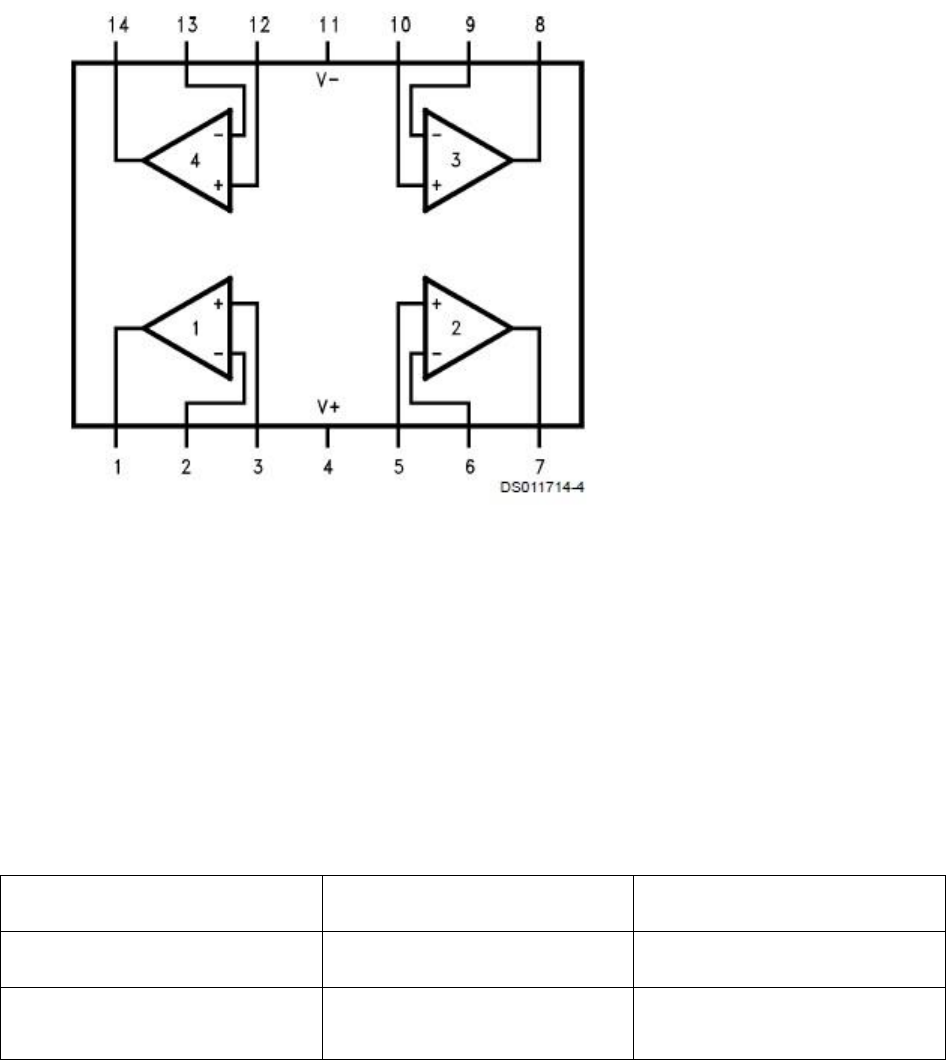LMC6484 Op-Amp Configuration is the one operational amplifier used in this lab to test the
amplification of an input signal to the amplification of this voltage signal, and the results are
tested in the output of the operational amplify.
In this procedure, the voltages were measured without using the amplifier using an oscilloscope
in CH1 and CH2 where the results from the lab experiment were as shown below in the table
below:
Expected Voltage at Ch1+
Measured Voltage at Ch1+
1MΩ Voltage Divider
2.5 V
1.722972155 V
499KΩ Voltage Divider
2.5 V
2.549085517 V
The results measured as shown in the table above were not amplified by the operational amplify.
After the 1MΩ Voltage Divider, the voltage decreased due to the high resistance and similar on
the other value measure the voltage almost remained as the resistance was very low. Using theop-amp shown above, the same experiment was repeated, and the results are as shown in the
table below.
Expected Voltage at Ch1+
Measured Voltage at Ch1+
1 MΩ Voltage Divider
2.5 V
2.757737038 V
499KΩ Voltage Divider
2.5 V
2.52256059 V
In this results, the measured voltages were amplified regardless of the high resistance voltage
divider. The op-amp shows some inverse proportionality in the two experiments.
Procedure B: Relationship between the output and input of an Op-amp
Measurement of Voltage for Voltage Divider Circuit without an Op-Amp Buffer. Connect two
1Mresistors in series. Connect 5V power source to pass through the resistors and ground the
other the other side of the resistor. Connect Ch1+ of the oscilloscope in between the two resistors
and ground it. Record the voltage as displayed in the display area of the oscilloscope. Repeat the
same procedure using 499kΩ resistors and record the readings. Measurement of Voltage for
Voltage Divider Circuit with an Op-Amp Buffer. Connect output pin of the 1mΩ voltage divider
to the non-inverting pin 3 of the OP-Amp Buffer. Connect 5v power to the Op-Amp Buffer by
connecting the positive terminal of the power source to pin 4 of the amplifier. Ground the
amplifier via pin 11. Short inverting pin 2 of the Op-Amp Buffer with output pin 1 Op-Amp
Buffer amplifier. Ground the oscilloscope and record the voltage reading as shown in the display.
Repeat the same procedure for 499kΩ and record the voltage readings. Draw a graph of this
representation to show the relationship between output voltage and input voltages of the op-amp.
The graph will have a negative slope as shown below.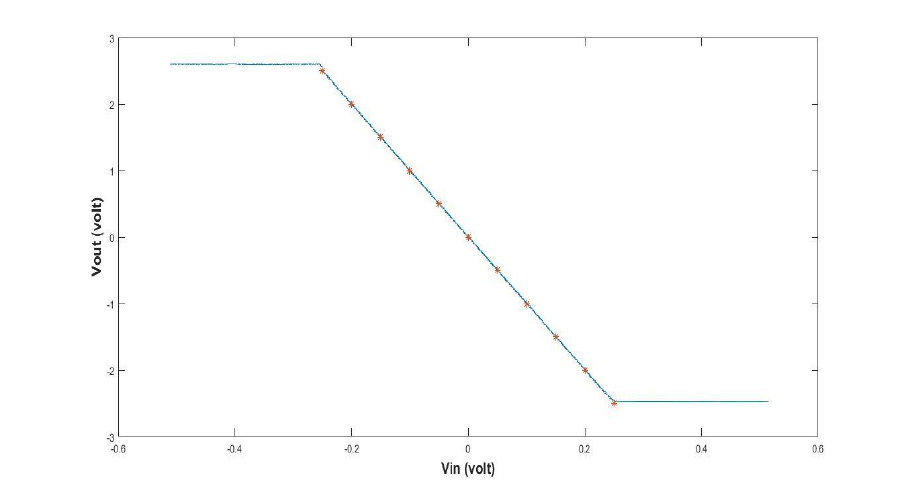Procedure C: Inverting amplifier circuit of the Op-amp
Use waveform Generator to create 100mV aptitude sine wave that has offset of 2.5V and
frequency of 1Hz and connected it to the circuit via pin 2 of the amplifier. Connect 10k resistor
in series in between the waveform generator and inverting pin 2 of the amplifier. Connect pin 3
of the amplifier to the 2.5V signal source. Connect a resistor of 10k between pin 2 and output pin
1 of the amplifier. Ground the amplifier via pin 11 and power it from 5V voltage source from pin
4. Then open the scope and use Ch1+ to measure the input and Ch2+ the output voltage. (Ch1-
and Ch2- should be placed at 2.5V.) Slowly increase the amplitude of the input waveform at
stopping at an interval of 5mV at keeping frequency constant and record the display signal at the
oscilloscope. The frequency filter can then be bode plotted as shown in the figure below.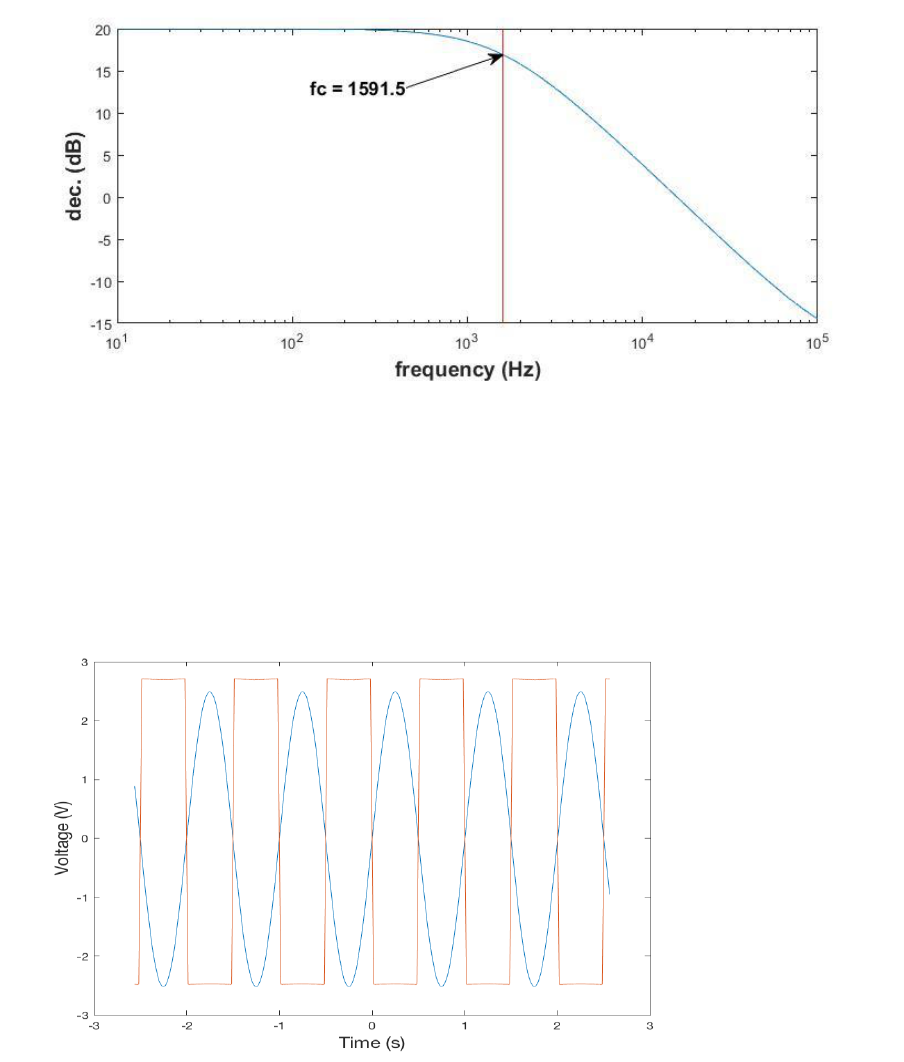The filter will result in the following voltage-time signals after being analyzed using the software
capable of filter analysis. Both waveforms are Inverting Amplifier Circuit waveform with ten
mV amplitude, 1 Hz frequency, and 2.5 V offset.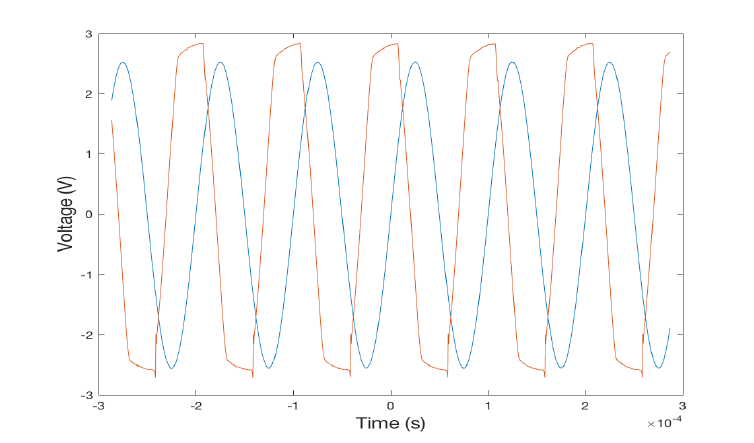Procedure D:
Inverting Amplifier Circuit waveform with ten mV amplitude, 10 kHz frequency and 2.5 V
offset Build the circuit similar to the inverting amplifier circuit and add a capacitor in across
inverting pin 2 and output pin 1 of the Op-Amp Buffer. Create a waveform which has a
waveform with ten mV amplitude, 1 Hz frequency, and 2.5 V offset. Analyse input ch1+ and
output ch2+ on the oscilloscope. Adjust voltage timescales to see a signal on the oscilloscope.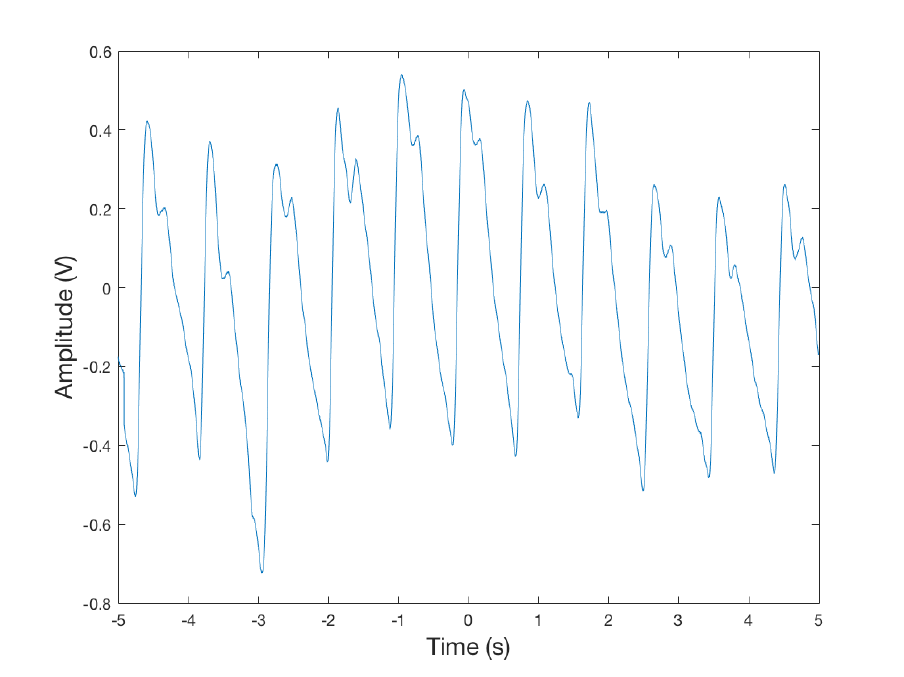Record the waveform displayed. The waveforms are as shown below.
III. Conclusions
From the procedure A of the op-amp experiment, we can conclude that the op-amp is an
electronic device that increases or amplifies the DC voltage. In the first set up using different
voltage dividers, the highest voltage divider gave a very low voltage in the measured value
which is true according to Kirchoff's voltage rule. This set up was not connected to the op-amp.
On connection of the op-amp, this voltage was inverse since the value measured under the high
resistance voltage divider was highly amplified than the other one as shown in the results.
The conclusion from procedure B. There was a slight drop in voltage measured at channel 1 of
the oscilloscope when 1 MΩ voltage divider was connected across the circuit. The voltage across
499 kΩ voltage divider increases slightly. The initial measured voltage in the channel was 2.5V.
When 1 MΩ voltage was connected, the measured voltage dropped to 1.722972155 V, and when
499kΩ voltage divider was connected voltage measured increased to 2.549085517 V., There was
lesser voltage recorded when using one mΩ voltage divider compared to 499 kΩ voltage divider.
The expected voltage to be measured in both connections of the 1mΩ and 499kΩ voltage
dividers when connected separately with Op-Amplifier Buffer was to be 2.5V. But when 1mΩ
voltage divider was connected with Op-Amplifier Buffer in the circuit, the measured voltage
from channel of the oscilloscope slightly increased to 2.757737038 V, and on connecting 499kΩ
in a similar manner as with 1mΩ, the voltage measured at channel 1 of the oscilloscope also
increased slightly to 2.52256059 V. In this case, the measured voltage when 499kΩ voltage
divider use was slightly lower than when using 1mΩ.
The conclusion from procedure C. The output decreases with increase in the input voltage
resulting to the graph with a slope of -10. Low pass filter with cut off frequency. Connect 10kΩ
capacitor in parallel with 10k resistor to circuit similar to one described in inverting amplifier
circuit above. Keeping ch1+ and ch2+ in the same place creates a Bode Plot network analyzers,
vary frequency from 10 to 10000Hz, amplitude of 10Mv and 2.5 V. Develop a waveform with
10mV and compare input ch1+ and output ch2+ on the oscilloscope. The waveform is as shown
above in the results of the procedure.
The conclusion from procedure D. It shows a waveform formed from the amplification of the
voltages as measure from an operational amplifier using an oscilloscope. This can make the
conclusion that the op-amp is useful for amplifying small voltage values to make them to a
useful one which is mainly required to be used in an electronic circuit.References
 Horowitz, Paul; Hill, Winfield (1989). The Art of Electronics. Cambridge, UK: Cambridge
University Press.
 Jung, Walter G. (2004). "Chapter 8: Op Amp History". Op Amp Applications Handbook.
Newnes. p. 77
 Jimmie J. Cathey 2008, Schaum’s Outline of Theory and Problems of Electronic Devices and
Circuits, McGraw-Hill, New York, 2
nd
Ed.
 Mehta V.K. & Mehta R. 1993, Principles of Electronics, S. Chand, New Delhi, INDIA

-+
550 words

#### Our customers say##### Jeff Curtis
USA, Student

"I'm fully satisfied with the essay I've just received. When I read it, I felt like it was exactly what I wanted to say, but couldn’t find the necessary words. Thank you!"##### Ian McGregor
UK, Student

"I don’t know what I would do without your assistance! With your help, I met my deadline just in time and the work was very professional. I will be back in several days with another assignment!"##### Shannon Williams

"It was the perfect experience! I enjoyed working with my writer, he delivered my work on time and followed all the guidelines about the referencing and contents."

• 5-paragraph Essay
• Annotated Bibliography
• Argumentative Essay
• Article Review
• Assignment
• Biography
• Book/Movie Review
• Case Study
• Cause and Effect Essay
• Classification Essay
• Comparison Essay
• Coursework
• Creative Writing
• Critical Thinking/Review
• Deductive Essay
• Definition Essay
• Essay (Any Type)
• Exploratory Essay
• Expository Essay
• Informal Essay
• Literature Essay
• Multiple Choice Question
• Narrative Essay
• Personal Essay
• Persuasive Essay
• Powerpoint Presentation
• Reflective Writing
• Research Essay
• Response Essay
• Scholarship Essay
• Term Paper
We use cookies to provide you with the best possible experience. By using this website you are accepting the use of cookies mentioned in our Privacy Policy.